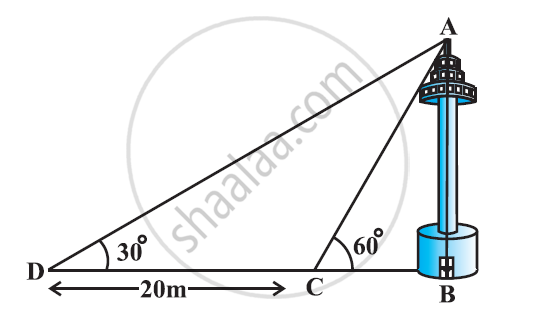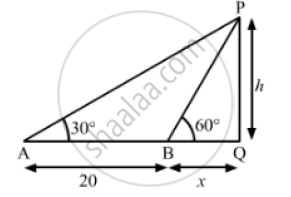# A TV tower stands vertically on a bank of a canal. From a point on the other bank directly opposite the tower the angle of elevation of the top of the tower is 60°. Find the height of the tower. - Mathematics

A TV tower stands vertically on a bank of a canal. From a point on the other bank directly opposite the tower the angle of elevation of the top of the tower is 60°. From another point 20 m away from this point on the line joining this point to the foot of the tower, the angle of elevation of the top of the tower is 30°. Find the height of the tower and the with of the canal.#### Solution 1

In ΔABC,

AB/BC = tan 60º

(AB)/(BC) = sqrt3

BC = (AB)/sqrt3

In ΔABD,

AB/BD =tan 30º

(AB)/(BC+CD) = 1/sqrt3

(AB)/((AB)/sqrt3+20) = 1/sqrt3

(ABsqrt3)/(AB+20sqrt3) = 1/sqrt3

3AB = AB+20sqrt3

2AB =  20sqrt3

AB = 10sqrt3 m

BC = (AB)/sqrt3 = ((10sqrt3)/sqrt3)m = 10 m

Therefore, the height of the tower is 10sqrt3  m and the width of the canal is 10 m.

#### Solution 2Let PQ=h m be the height of the TV tower and BQ= x m be the width of the canal.
We have,
AB = 20 m, ∠PAQ = 30° ,∠BQ = x and PQ  = h
In ΔPBQ,

 tan 60° = (PQ)/(BQ)

⇒ sqrt(3) = h/x

⇒ h = x sqrt(3)                 ........................(1)

Again in ΔAPQ,

tan 30° = (PQ)/(AQ)

⇒ 1/sqrt(3) = h/(AB +BQ)

⇒ 1/sqrt(3) = (x sqrt(3)) / (20+3)                       [ Using (1)]

⇒ 3x = 20+x

⇒3x -x =20

⇒ 2x = 20

⇒ x=20/2

⇒ x = 10 m

Substituting x = 10 in (i),we get
h = 10 sqrt(3)m
So, the height of the TV tower is 10sqrt( 3) mand the width of the canal is 10 m.

Concept: Heights and Distances
Is there an error in this question or solution?
Chapter 9: Some Applications of Trigonometry - Exercise 9.1 [Page 204]

#### APPEARS IN

NCERT Class 10 Maths
Chapter 9 Some Applications of Trigonometry
Exercise 9.1 | Q 11 | Page 204
RS Aggarwal Secondary School Class 10 Maths
Chapter 14 Height and Distance
Exercises | Q 15

Share